# A Different Sense of Distance

There are other ways to compare rational numbers, especially if one happens to enjoy number theory...

# A Different Sense of Distance

Maria Fox
Oklahoma State University

Dedicated to my Dad, Dr. Barry R. Fox.

The idea of distance is central to so much of the mathematics we do and teach that it's easy not to give it a second thought. But what do we mean when we say two rational numbers are "close" or "far apart"? For example, how do we decide if $\frac{7718}{23029}$ or $\frac{6056}{18143}$ is closer to $\frac{1}{3}$?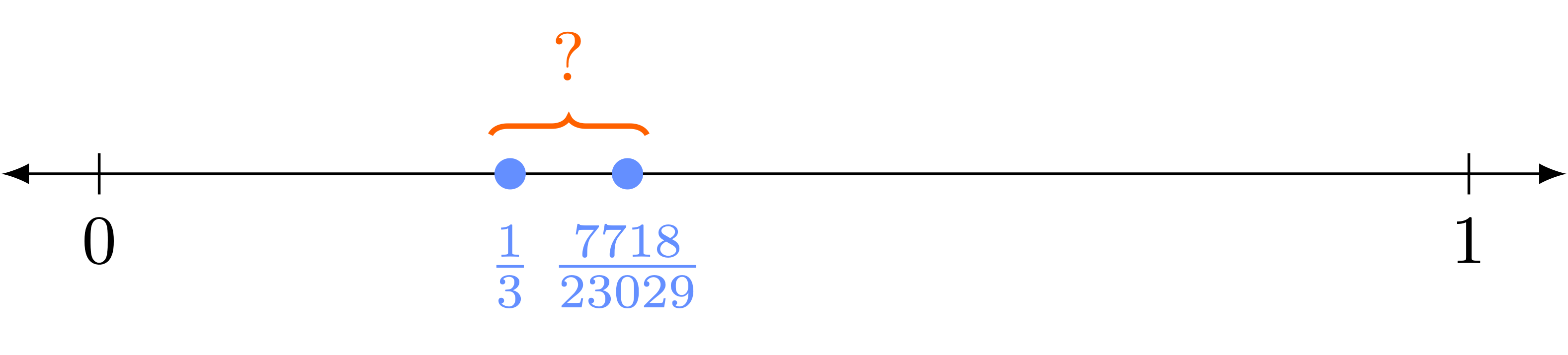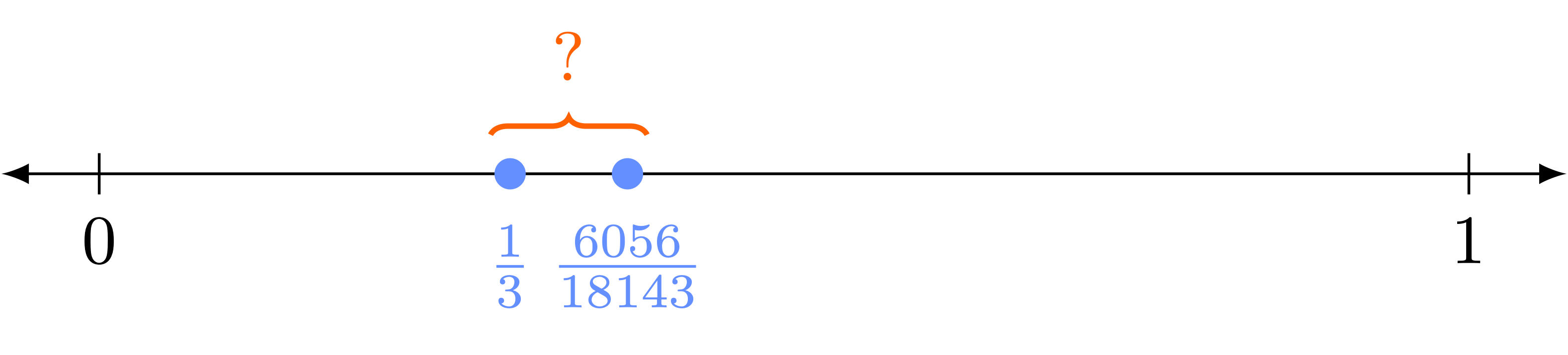A common approach is to compare the decimal expansion of $\frac{1}{3} = 0.33333...$ with the expansions of these two numbers:
$$\frac{7718}{23029} = 0.33514264... \quad \quad \frac{6056}{18143} = 0.33379264...$$

From these expansions, both rational numbers seem quite close to $\frac{1}{3}$. Indeed, if we truncated all three numbers at the tenths place, they would be indistinguishable: all equal to 0.3. Even at the hundredths place, they still agree. It's only when we consider the thousandths place that we can tell them apart: truncating to 0.333, the rational number $\frac{6056}{18143}$ continues to agree with $\frac{1}{3}$, while $\frac{7718}{23029}$ truncates to 0.335 and does not.

This is an excellent way to understand our usual distance function: as we compute further and further decimal expansions of two rational numbers $\frac{a}{b}$ and $\frac{c}{d}$, the longer they agree, the smaller the distance between them. To separate this idea from alternative notions of distance, we'll refer to this usual type of distance as the Archimedean distance, defined as $d_\mathrm{Arch}(\frac{a}{b}, \frac{c}{d} ) = \sqrt{ (\frac{a}{b} - \frac{c}{d})^2 }$.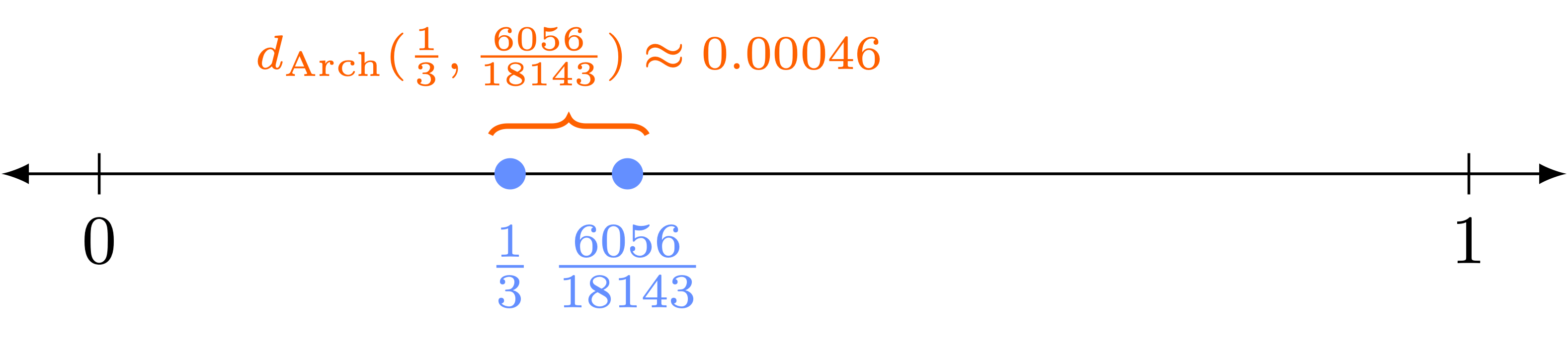Under the Archimedean distance, $\frac{6056}{18143}$ is closer to $\frac{1}{3}$ than $\frac{7718}{23029}$ is.

But there are other ways to compare rational numbers, especially if one happens to enjoy number theory. We can consider their reductions modulo a prime, for example $5$. Whenever $5$ does not divide the denominator of a rational number $\frac{a}{b}$ (which we'll assume for now), then $b$ has a multiplicative inverse modulo $5$, and we can use this inverse to make sense of $\frac{a}{b}$ modulo 5.

In particular, since $3 \cdot 2 \equiv 1 \pmod 5$, 2 is a multiplicative inverse of 3 modulo 5. Using this, we can define $\frac{1}{3}$ modulo 5 as $1 \cdot 3^{-1} \equiv 2 \pmod 5$. Similarly, we can view both $\frac{6056}{18143}$ and $\frac{7718}{23029}$ modulo 5:

\begin{align*}
\frac{7718}{23029} &\equiv 7718 \cdot 23029^{-1} &\pmod 5 \\
& \equiv 7718 \cdot 4 &\pmod 5 \\
& \equiv 30872 &\pmod 5 \\
&\equiv 2 &\pmod 5 \\
\end{align*}

\begin{align*}
\frac{6056}{18143} &\equiv 6056 \cdot 18143^{-1} &\pmod 5 \\
& \equiv 6056 \cdot 2 &\pmod 5 \\
& \equiv 12112 &\pmod 5 \\
&\equiv 2 &\pmod 5 \\
\end{align*}

Interestingly, both $\frac{6056}{18143}$ and $\frac{7718}{23029}$ agree with $\frac{1}{3}$ modulo 5. That is, if we use "reduction modulo 5" as a first glance at these competing rational numbers, they are both indistinguishable from $\frac{1}{3}$.

But we can take this a step further and compare these rational numbers modulo $5^2 = 25$. Since 5 does not divide the denominators, it is possible to compute the necessary multiplicative inverses and reduce these rational numbers modulo 25:

\begin{align*}
\frac{7718}{23029} &\equiv 7718 \cdot 23029^{-1} &\pmod{25} \\
&\equiv 7718 \cdot 19 &\pmod{25} \\
& \equiv 17 &\pmod{25} \\
\end{align*}

\begin{align*}
\frac{6056}{18143} & \equiv 6065 \cdot 18143^{-1} &\pmod{25} \\
&\equiv 6056 \cdot 7 &\pmod{25} \\
& \equiv 17 &\pmod{25}. \\
\end{align*}

Again, they both agree with $\frac{1}{3} \equiv 17 \pmod{25}$.

Moving on to the next power of 5, we can finally see a difference when comparing $\frac{1}{3} \equiv 42 \pmod{125}$ and:
$$\frac{7718}{23029} \equiv 42 \pmod{125} \quad \quad \frac{6056}{18143} \equiv 17 \pmod{125}.$$
Viewed modulo 125, the rational number $\frac{7718}{23029}$ continues to agree with $\frac{1}{3}$, while $\frac{6056}{18143}$ does not. In other words, using our reduction-modulo-powers-of-5 technique, it takes longer to distinguish $\frac{7718}{23029}$ from $\frac{1}{3}$ than it takes to distinguish $\frac{6056}{18143}$ from $\frac{1}{3}$. In this sense, $\frac{7718}{23029}$ is closer to $\frac{1}{3}$ than $\frac{6056}{18143}$ is.

This is the central idea behind the 5-adic distance. Assuming that $5$ does not divide the denominators of $\frac{a}{b}$ or $\frac{c}{d}$, we define:
$$d_5 \left( \frac{a}{b}, \frac{c}{d} \right) = \left( \frac{1}{5} \right)^{\nu( \frac{a}{b} - \frac{c}{d} )},$$
where $\nu( \frac{a}{b} - \frac{c}{d} )$ is the number of times 5 appears in the prime factorization of the numerator of $\frac{a}{b} - \frac{c}{d} = \frac{ad-bc}{bd}$, or equivalently the largest integer $n$ for which $\frac{a}{b}$ and $\frac{c}{d}$ agree modulo $5^n$. This exactly captures our approach above: as we compare $\frac{a}{b}$ and $\frac{c}{d}$ modulo $5, \ 5^2, \ 5^3, \ 5^4,$ etc., the longer these rational numbers agree, the smaller the 5-adic distance $d_5( \frac{a}{b}, \frac{c}{d} )$ between them.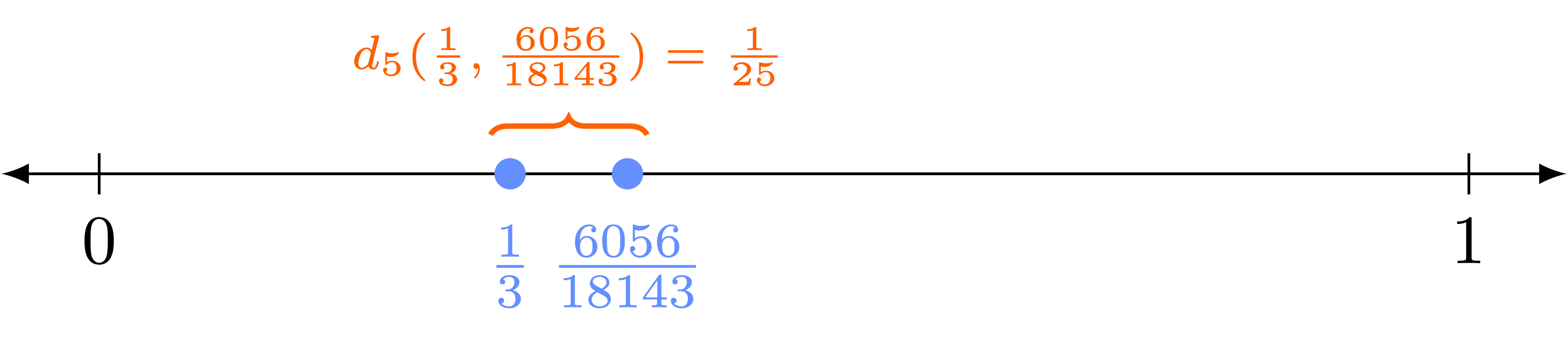In particular, under the 5-adic distance, $\frac{7718}{23029}$ is closer to $\frac{1}{3}$ than $\frac{6056}{18143}$ is. (Notice that this is the opposite of our conclusion under the Archimedean distance!)

The 5-adic distance function behaves very differently than the Archimedean distance. For example the integers $5, 25, 125, 625, ...$ grow closer and closer to 0 under the 5-adic distance, but farther and farther from 0 under the Archimedean distance. If we extend the 5-adic distance in a natural way to allow 5 to appear in the denominators of rational numbers (in this case, we define $\nu(\frac{a}{b}-\frac{c}{d})$ to be $-n$, where $n$ is the number of times 5 appears in the prime factorization of the denominator of $\frac{a}{b} - \frac{c}{d} = \frac{ab-bc}{bd}$), then $\frac{1}{5}, \frac{1}{25}, \frac{1}{125}, \frac{1}{625}$, ... will grow farther and farther from 0 in the 5-adic distance, but closer and closer to 0 under the Archimedean distance.

## Effects on Triangles

Now that we have a new type of distance, what is the effect on some familiar geometric objects? As a first example, we'll consider how familiar properties of triangles suddenly change under the 5-adic distance.

First, we need to adjust our idea of triangles. Triangles are most commonly considered in 2-dimensional space, as in the image below.But we can just as easily consider triangles in 1-dimensional space! We'll define a triangle inside the rational numbers to simply be a choice of its three vertices: that is, a choice of three rational numbers $\frac{a}{b}$, $\frac{c}{d}$, and $\frac{e}{f}$. The side lengths are then the distances between the three possible pairs of vertices. For example, below is an illustration of the triangle with vertices $\frac{1}{2}$, $\frac{7}{3}$, and $\frac{21}{4}$.Triangles enjoy the familiar Triangle Inequality under the Archimedean distance: each side length is less than or equal to the sum of the other two side lengths:
$$d_\mathrm{Arch}\left(\frac{a}{b}, \frac{c}{d} \right) \leq d_\mathrm{Arch} \left(\frac{a}{b}, \frac{e}{f} \right) + d_\mathrm{Arch} \left(\frac{c}{d}, \frac{e}{f} \right).$$
This is true regardless of the way the vertices are labelled. After computing the side lengths of this triangle under the Archimedean distance,our example above meets three Triangle Inequalities (depending on the labelling of the vertices):

\begin{align*}
d_\mathrm{Arch} \left(\frac{1}{2}, \frac{7}{3} \right) &\leq d_\mathrm{Arch} \left(\frac{1}{2}, \frac{21}{4} \right) + d_\mathrm{Arch} \left(\frac{7}{3}, \frac{21}{4} \right) &1.8\overline{3} < 4.75 + 2.91\overline{6} \\
d_\mathrm{Arch} \left(\frac{1}{2}, \frac{21}{4} \right) &\leq d_\mathrm{Arch} \left(\frac{1}{2}, \frac{7}{3} \right) + d_\mathrm{Arch} \left(\frac{21}{4}, \frac{7}{3} \right) &4.75 = 1.8\overline{3} + 2.91\overline{6} \\
d_\mathrm{Arch} \left(\frac{21}{4}, \frac{7}{3} \right) &\leq d_\mathrm{Arch} \left(\frac{21}{4}, \frac{1}{2} \right) + d_\mathrm{Arch} \left(\frac{7}{3}, \frac{1}{2} \right) &2.91\overline{6} < 4.75 + 1.8\overline{3}.
\end{align*}

But what happens under the 5-adic distance? Now our triangle has 5-adic side lengths as labeled below:In fact, triangles in the 5-adic distance enjoy the even stronger Non-Archimedean Triangle Inequality:
$$d_5 \left(\frac{a}{b}, \frac{c}{d} \right) \leq \mathrm{max} \left\{ d_5 \left(\frac{a}{b}, \frac{e}{f} \right), \ d_5 \left(\frac{c}{d}, \frac{e}{f} \right) \right\},$$
regardless of the way the vertices are labelled. So, we have the three Non-Archimedean Triangle Inequalities from this example:
\begin{align*}
d_5 \left(\frac{1}{2}, \frac{7}{3} \right) &\leq \mathrm{max} \left\{ d_5 \left(\frac{1}{2}, \frac{21}{4} \right), \ d_5 \left(\frac{7}{3}, \frac{21}{4} \right) \right\} &1 = \mathrm{max} \Bigl\{1, \frac{1}{5} \Bigr\} \\
d_5 \left(\frac{1}{2}, \frac{21}{4} \right) &\leq \mathrm{max} \left\{ d_5 \left(\frac{1}{2}, \frac{7}{3} \right), \ d_5 \left(\frac{21}{4}, \frac{7}{3} \right) \right\} &1 = \mathrm{max} \Bigl\{ 1, \frac{1}{5} \Bigr\} \\
d_5 \left(\frac{21}{4}, \frac{7}{3} \right) &\leq \mathrm{max} \left\{ d_5 \left(\frac{21}{4}, \frac{1}{2} \right), \ d_5 \left(\frac{7}{3}, \frac{1}{2} \right) \right\} &\frac{1}{5} < \mathrm{max} \Bigl\{ 1, 1 \Bigr\}.
\end{align*}

Unlike under the Archimedean metric, this triangle is "isosceles" under the 5-adic metric: two of its side lengths are equal. This is not an accident! Just as we saw in this example, whenever $d_5(\frac{a}{b}, \frac{e}{f}) \neq d_5(\frac{c}{d}, \frac{e}{f})$, the third length $d_5(\frac{a}{b}, \frac{c}{d})$ is exactly equal to their maximum. As a result,

### Theorem.

All triangles are isosceles under the 5-adic distance.

Given any three rational numbers $\frac{a}{b}$, $\frac{c}{d}$, $\frac{e}{f}$, at least two of the three distances $d_5(\frac{a}{b}, \frac{c}{d})$, $d_5(\frac{a}{b}, \frac{e}{f})$, and $d_5(\frac{c}{d}, \frac{e}{f})$ will be equal.

This is certainly different from triangles under the Archimedean distance!

## Effects on Circles

Circles also behave quite differently under the 5-adic distance. As before, even though it is most common to consider circles in 2-dimensional or 3-dimensional space—as illustrated below—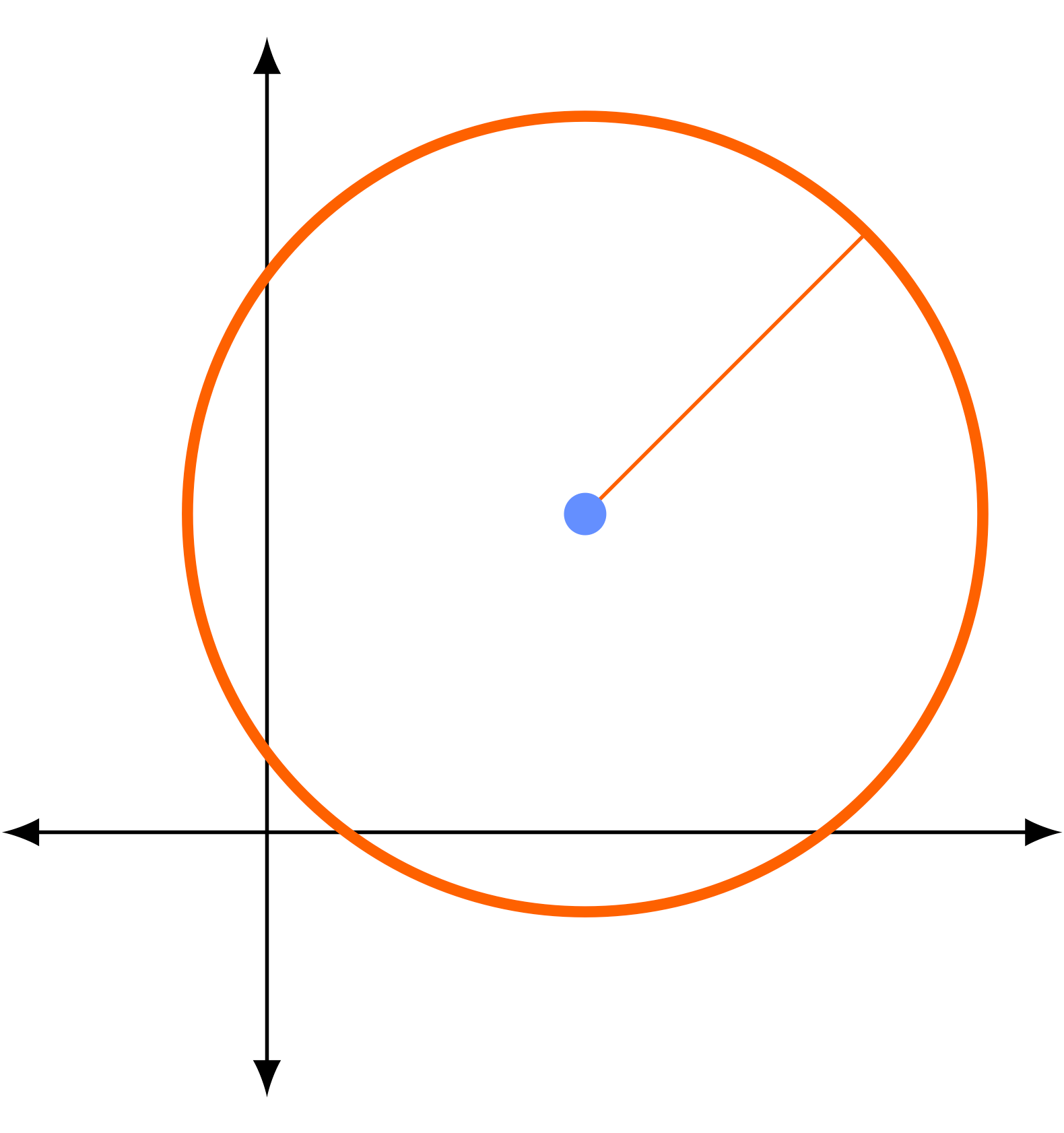we will consider circles in 1-dimensional space. A circle in the rational numbers is determined by a center $\frac{a}{b}$ and a radius $r$. The circle $C(\frac{a}{b}, r)$ of radius $r$ about the point $\frac{a}{b}$ is defined to be all those rational numbers whose distance from $\frac{a}{b}$ is strictly less than $r$. (This is an "open circle," though we could have just as easily studied "closed circles" by allowing the distance to also be equal to $r$.)

For example, let $C_{\mathrm{Arch}}(\frac{7}{3}, 2)$ be the circle of radius 2 about $\frac{7}{3}$, under the Archimedean distance:
$$C_\mathrm{Arch}\left( \frac{7}{3}, 2 \right) = \left\{ \frac{a}{b} \ \middle| \ d_\mathrm{Arch}\left( \frac{7}{3}, \frac{a}{b} \right) < 2 \right\}.$$
From the definition of Archimedean distance, we can identify $C_\mathrm{Arch}( \frac{7}{3}, 2)$ with the open interval between $\frac{1}{3}$ and $\frac{13}{3}$:Unfortunately, it's much more difficult to draw a circle under the 5-adic distance! For example, consider the circle $C_{5}(\frac{7}{3}, 2)$ of radius 2 about $\frac{7}{3}$, under the 5-adic distance:
$$C_5 \left( \frac{7}{3}, 2 \right) = \left\{ \frac{a}{b} \ \middle| \ d_5 \left( \frac{7}{3}, \frac{a}{b} \right) < 2 \right\}.$$
The first thing to notice is that the 5-adic distance between any two rational numbers will always be a power of 5 (including negative powers, like $\frac{1}{5}$). So requiring the 5-adic distance to be less than 2 is the same as requiring the 5-adic distance to be less than or equal to 1.

Whenever 5 does not divide $b$, the distance $d_5(\frac{7}{3}, \frac{a}{b})$ between $\frac{7}{3}$ and $\frac{a}{b}$ will always be at most 1, because the exponent $\nu(\frac{7}{3} - \frac{a}{b})$ will be positive or zero. So, for example, the rational numbers $-\frac{7}{8}$, $\frac{2}{3}$, $2$, and $\frac{9}{2}$ are all contained in this circle: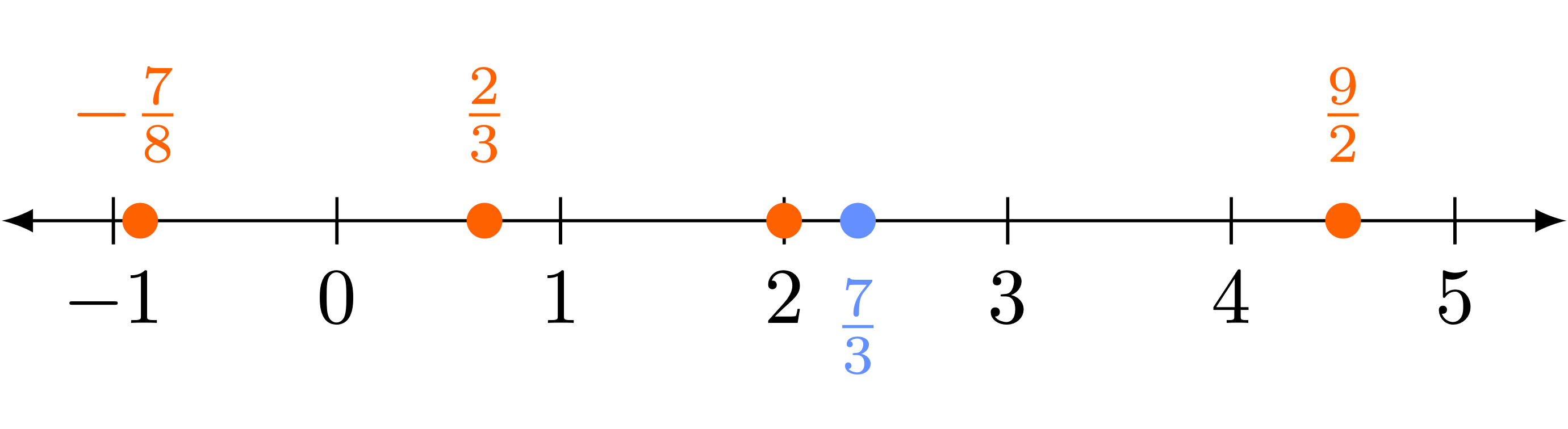But so are infinitely more rational numbers. In fact, every single integer will be contained in this circle:It's also illustrative to consider some points that do not lie in this circle. Both $-\frac{1}{5}$ and $\frac{8}{5}$ are 5-adic distance 5 from $\frac{7}{3}$, the rational number $\frac{14}{25}$ is distance 25 from $\frac{7}{3}$, and $\frac{401}{125}$ is distance $125$ from $\frac{7}{3}$. So, none of these points are contained in the circle $C_5( \frac{7}{3}, 2)$: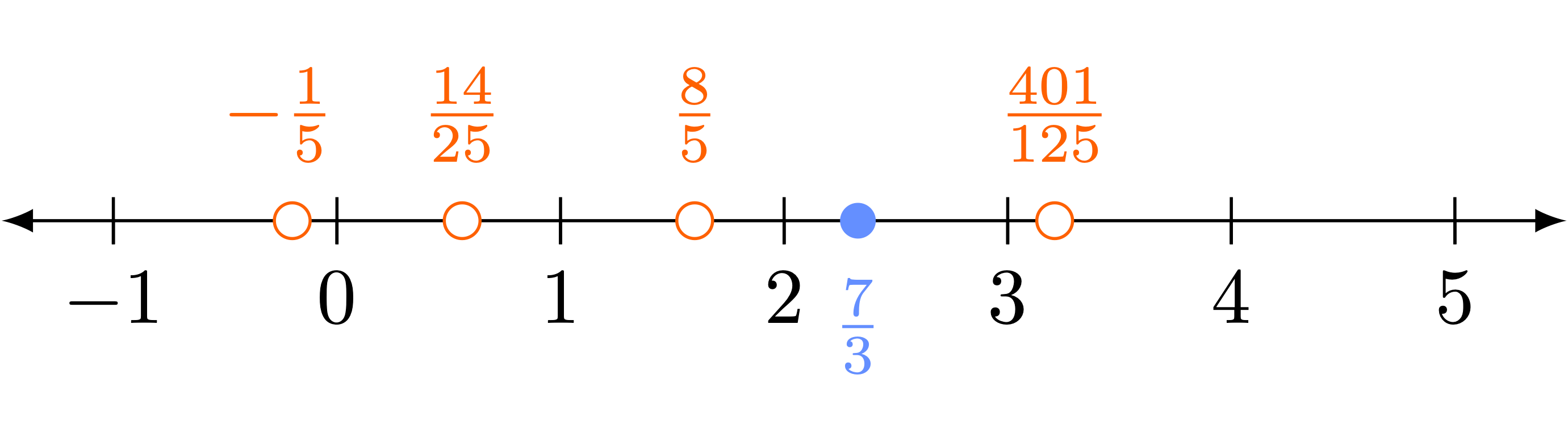Not only are 5-adic circles challenging to draw, they also behave very differently from Archimedean circles. For example, using the fact that all 5-adic triangles are isosceles, one can show the following:

### Theorem.

Any point in a 5-adic circle can be used as the center of the circle.

Whenever $\frac{c}{d} \in C_5( \frac{a}{b}, r)$, then the circles of radius $r$ about $\frac{a}{b}$ and $\frac{c}{d}$ are equal: $C_5( \frac{a}{b}, r) = C_5( \frac{c}{d}, r).$

As an example, since $-\frac{7}{8}$, $\frac{2}{3}$, $2$, and $\frac{9}{2}$ are all contained in $C_5( \frac{7}{3}, 2),$ the circles $C_5( -\frac{7}{8}, 2),$ $C_5( \frac{2}{3}, 2),$ $C_5( 2, 2),$ and $C_5( \frac{9}{2}, 2),$ are all equal to our original circle $C_5( \frac{7}{3}, 2)$.

As a result of the above theorem, we have another surprising fact about 5-adic circles:

### Theorem.

Any two 5-adic circles are disjoint or concentric.

If the intersection of $C_5( \frac{a}{b}, r)$ and $C_5( \frac{c}{d}, t)$ is nonempty, then the circle with the smaller radius (without loss of generality, $t$) is contained in the circle with the larger radius: $C_5( \frac{c}{d}, t) \subseteq C_5( \frac{a}{b}, r).$

This is very different from Archimedean circles. For example, $C_{\mathrm{Arch} }( \frac{1}{2}, 1.3)$ and $C_\mathrm{Arch}( \frac{8}{3}, 1.5)$ are two non-concentric, non-disjoint Archimedean circles:This never occurs under the 5-adic distance!

## Effects on Manifolds

The surprising geometric properties of the 5-adic distance that we have already noticed have far-reaching effects. The notion of Archimedean distance is the starting point for the study of real manifolds: the real numbers $\mathbb{R}$ can be constructed as the completion of the rational numbers under the Archimedean distance. Then, real manifolds are defined to be topological spaces that look like $\mathbb{R}$ (or $\mathbb{R}^2$, or $\mathbb{R}^3$, ...) around every point, once one zooms in far enough. Below are illustrations of some 2-dimensional real manifolds: a Klein bottle and a torus.Image from Wikimedia Commons (CC BY-SA 3.0)Image from Wikimedia Commons (CC BY-SA 3.0)

What happens when we try to repeat this construction with the 5-adic distance instead of the Archimedean distance? That is, how can we create 5-adic manifolds? First, one can replicate the process of constructing the real numbers from the rational numbers, but with the 5-adic distance in place of the Archimedean distance. This produces the 5-adic numbers, $\mathbb{Q}_5$, and if 5 is replaced with another prime $p$ this construction will yield the $p$-adic numbers, $\mathbb{Q}_p$.

One possible construction of $p$-adic manifolds (the theory of $\mathbb{Q}_p$-analytic spaces, as described by Jean-Pierre Serre in Lie groups and Lie algebras) is very analogous to the construction of real manifolds. While the natural approach in some circumstances, $\mathbb{Q}_p$-analytic spaces leave also some things to be desired. Without getting into the technicalities, these inconveniences can be summed up perfectly in the words of John Tate, who referred to $\mathbb{Q}_p$-analytic spaces as "wobbly" analytic spaces!

This wobbliness fundamentally originates from our third observation about the $p$-adic distance: any two $p$-adic circles are disjoint or concentric. Because of this, there are many ways to write one open circle as a union of others (called an "open cover"). To fix the wobbliness of $\mathbb{Q}_p$-analytic spaces, there have been two main approaches. The first, pioneered by Tate in the 1960s (and then developed by many others) used ideas of Alexander Grothendieck to formally disallow some open covers. These $p$-adic manifolds are called rigid analytic spaces (in contrast with the original wobbly ones).

A second approach was developed in the 1990s by Roland Huber, who removed the wobbliness of $\mathbb{Q}_p$-analytic spaces essentially by adding in some "missing points." These $p$-adic manifolds are called adic spaces. Perfectoid spaces, powerful tools in number theory and algebraic geometry recently developed by Peter Scholze, are a special kind of adic space. In this sense, our geometric observations about triangles and circles under the 5-adic distance are the root of cutting-edge mathematical developments today.

## Which Distance is More Natural?

Just because the 5-adic distance enjoys many different properties from the Archimedean distance, it doesn't mean that this idea of distance is either new or unusual.

The Archimedean distance is undoubtably older: it is named for the "Archimedean property" of this distance function, and Archimedes himself originally named this property after Eudoxus of Cnidus (who was born around 400 BC). However, the $5$-adic distance is also very old. The general concept of a $p$-adic distance (defined analogously to the 5-adic distance, for any prime $p$) was first formally studied by Kurt Hensel in his 1897 paper Über eine neue Begründung der Theorie der algebraischen Zahlen. Related ideas can be traced back at least a little further to the work of Ernst Kummer.

Additionally, by Ostrowski's Theorem, the only distance functions on the rational numbers are the Archimedean distance $d_\mathrm{Arch}$ and the $p$-adic distances $d_p$. Since there are infinitely many $p$-adic distance functions and only one Archimedean one, perhaps the Archimedean distance should be considered as the "unusual" one!

## Further Reading on $p$-adic Distance and $p$-adic Numbers

• A. I. Borevich and I. R. Shafarevich. Number theory. Pure and Applied Mathematics, Vol. 20. Academic Press, New York-London, 1966. Translated from the Russian by Newcomb Greenleaf
• Fernando Q. Gouvêa. $p$-adic numbers. Universitext. Springer, Cham, . Third edition.
• Svetlana Katok. $p$-adic analysis compared with real, volume 37 of Student Mathematical Library. American Mathematical Society, Providence, RI; Mathematics Advanced Study Semesters, University Park, PA, 2007
• Neal Koblitz. $p$-adic numbers, $p$-adic analysis, and zeta-functions, volume 58 of Grad-
uate Texts in Mathematics. Springer-Verlag, New York, second edition, 1984
• $p$-adic number and $p$-adic valuation on Wikipedia.

## Further Reading on $p$-adic Manifolds

• Bhargav Bhatt, Ana Caraiani, Kiran S. Kedlaya, and Jared Weinstein. Perfectoid spaces, volume 242 of Mathematical Surveys and Monographs. American Mathematical Society, Providence, RI, 2019. Lectures from the 2017 Arizona Winter School, held in Tucson, AZ, March 11-17, Edited and with a preface by Bryden Cais, With an introduction by Peter Scholze
• Bhargav Bhatt, What Is... A Perfectoid Space?, Notices of the AMS, October 2014.
• Siegfried Bosch. Lectures on formal and rigid geometry, volume 2105 of Lecture Notes in Mathematics. Springer, Cham, 2014
• Jean-Pierre Serre. Lie algebras and Lie groups, volume 1500 of Lecture Notes in Mathematics. Springer-Verlag, Berlin, 2006. 1964 lectures given at Harvard University, Corrected fifth printing of the second (1992) edition
• John Tate. Rigid analytic spaces. Invent. Math., 12:257-289, 1971